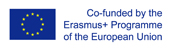This project has been funded with support from the European Commission.
This web site reflects the views only of the author, and the Commission cannot be held responsible for any use which may be made of the information contained therein.

Select language   >   IT EN RO PL LT

### Why is Pythagorean theorem important to the world of Math?

Subject taught
- Math

Language Skills Developed
- Interaction
- Listening
- Speaking

Transferable/Scientific Skills Developed
The resources helps students to develop their self–directed learning and problem solving skills while learning to understand what Pythagorean theorem is and how it can be used in real life situations.

Description
General aims
Students will learn key vocabulary in English and know how to:
- Define the Pythagorean theorem
- Solve equations using the Pythagorean Theorem
- Apply the Pythagorean theorem to determine unknown side lengths in right triangles in real-world and mathematical problems in two and three dimensions.

Linguistic aims
Basic English vocabulary linking with mathematical and technical terms.

Key words: Pythagorean Theorem, Pythagoras Theorem
Hypotenuse, triangle, angle, right-angle triangle, square root, leg, side, equation, formula

Subject specific aims:
Upon completion of this lesson, students should be able to:
- Define the Pythagorean theorem
- Solve equations using the Pythagorean Theorem
- Know key vocabulary in English

Target group age: lower secondary school students (13-14 years old).

Level of competence in English (CEFR) B1/B1+

Time required to use the resources with the students: 2-3 lessons

Firstly, teachers need to select and present vocabulary items that learners need to know and understand in order to be able to process the input.
Next, teachers can use the videos and discuss the content with students in English.
Then, it is a good time for doing exercises Clil 4 Steam (pixel-online.org), Clil 4 Steam (pixel-online.org) and Clil 4 Steam (pixel-online.org) - this is a cloze exercise which asks students to complete sentences by inserting the missing words. Students work in pairs and discuss answers in English.
Depending on time teacher can introduce more exercises presented on website Clil4Steam.pixel-online.org and as a homework students can do some exercises from the project website.

Possible difficulties for the students
Apply the Pythagorean theorem in real-world problems.

Teaching Resources (created)

Teaching Resources (reviewed)